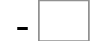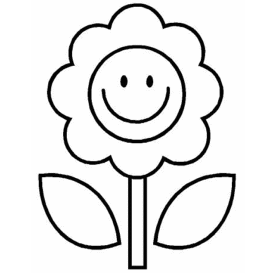70= 41   80= 1510= 0   16= 6   38= 13   75= 36 64= 16   81= 66   58= 26   17= 4